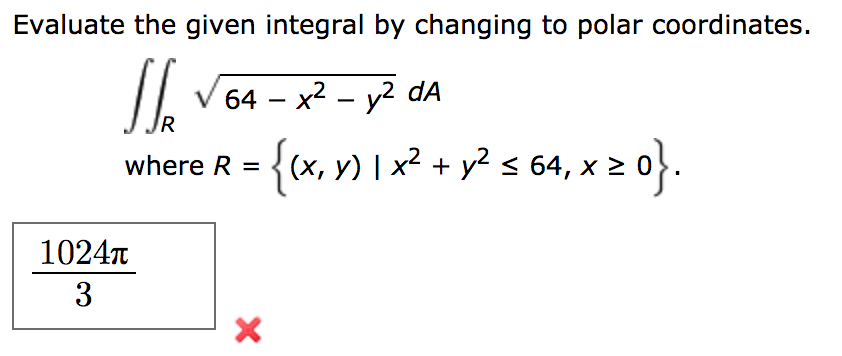# Evaluate the given integral by changing to polar coordinates.64 x2 - y2 dARwhere R =(x, y) I x2 + y2 s 64, x 21024tX

Question
3 viewshelp_outlineImage TranscriptioncloseEvaluate the given integral by changing to polar coordinates. 64 x2 - y2 dA R where R =(x, y) I x2 + y2 s 64, x 2 1024t X fullscreen
check_circle

Step 1

x2 + y2 ≤ 64 = r2

Hence, r = 8

Under polar coordinates: x = rcosƟ; y = r sinƟ; dA = rdrdƟ

Step 2

The region R is semi circle of radius 8 lying in the first and the second quadrant such that x ≥ 0

Hence limits of integration will be from Ɵ = -π/2 to Ɵ = π/2  and r = 0 to 8

Step 3

If the desired area is A, then pease see the work...

### Want to see the full answer?

See Solution

#### Want to see this answer and more?

Solutions are written by subject experts who are available 24/7. Questions are typically answered within 1 hour.*

See Solution
*Response times may vary by subject and question.
Tagged in

### Integration